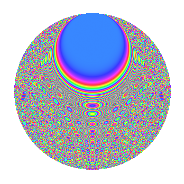# Properties

 Label 756.2.cfLevel 756 Weight 2 Character orbit cf Rep. character $$\chi_{756}(155,\cdot)$$ Character field $$\Q(\zeta_{18})$$ Dimension 648 Newform subspaces 1 Sturm bound 288 Trace bound 0

# Related objects

## Defining parameters

 Level: $$N$$ = $$756 = 2^{2} \cdot 3^{3} \cdot 7$$ Weight: $$k$$ = $$2$$ Character orbit: $$[\chi]$$ = 756.cf (of order $$18$$ and degree $$6$$) Character conductor: $$\operatorname{cond}(\chi)$$ = $$108$$ Character field: $$\Q(\zeta_{18})$$ Newform subspaces: $$1$$ Sturm bound: $$288$$ Trace bound: $$0$$

## Dimensions

The following table gives the dimensions of various subspaces of $$M_{2}(756, [\chi])$$.

Total New Old
Modular forms 888 648 240
Cusp forms 840 648 192
Eisenstein series 48 0 48

## Trace form

 $$648q + O(q^{10})$$ $$648q + 18q^{12} + 42q^{18} + 42q^{20} - 48q^{30} - 30q^{32} + 60q^{33} + 60q^{41} - 30q^{42} - 144q^{44} - 18q^{48} - 156q^{50} + 54q^{52} - 12q^{57} + 54q^{58} - 186q^{60} - 24q^{65} - 186q^{66} - 78q^{68} - 42q^{72} - 24q^{81} - 30q^{86} - 36q^{89} + 198q^{90} + 114q^{92} - 144q^{93} - 54q^{94} + 156q^{96} + 36q^{97} + O(q^{100})$$

## Decomposition of $$S_{2}^{\mathrm{new}}(756, [\chi])$$ into newform subspaces

Label Dim. $$A$$ Field CM Traces $q$-expansion
$$a_2$$ $$a_3$$ $$a_5$$ $$a_7$$
756.2.cf.a $$648$$ $$6.037$$ None $$0$$ $$0$$ $$0$$ $$0$$

## Decomposition of $$S_{2}^{\mathrm{old}}(756, [\chi])$$ into lower level spaces

$$S_{2}^{\mathrm{old}}(756, [\chi]) \cong$$ $$S_{2}^{\mathrm{new}}(108, [\chi])$$$$^{\oplus 2}$$

## Hecke Characteristic Polynomials

There are no characteristic polynomials of Hecke operators in the database